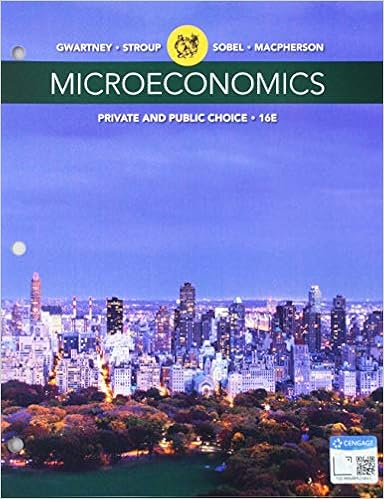Suppose the interest rate differential between dollar

• Homework Help
• 3

This preview shows page 3 out of 3 pages.

We have textbook solutions for you!
The document you are viewing contains questions related to this textbook.The document you are viewing contains questions related to this textbook.
Chapter 4 / Exercise 7
Microeconomics: Private and Public Choice
Gwartney/Stroup/Sobel/MacphersonExpert Verified
Suppose the interest rate differential between dollar and yen deposits drops to 2%. Explain what will happen to expected exchange rate and spot rate. 9. Given the following information: Spot rate of Swiss Franc …………………………………………….1 Frank=\$ 0.80 Forward rate of Swiss Franc …………………………… ……..…….1 Frank=\$ 0.79 Spot rate of Swiss Franc …………………………………………….1 Frank=TL 1.89 Forward rate of Swiss Franc …………………………… ……..…….1 Frank=TL 1.95 Interest rate on Swiss Franc deposits…… …………………………. 4 % Interest rate on US dollar deposits…………………..…………….…. 2.5 % Interest rate on Turkish Lira deposits…………………..…………….…. 5 % what rate of return can be earned on \$1m from covered interest arbitrage i) by a US investor and ii) by a Turkish investor? Is it worthwhile undertaking? 10 . Given the following data: Current US 1-year interest rate: 6% Current exchange rate \$1.00 = 0.90 Expected exchange rate one year from now: \$1.00 = 0.85 a) What is the equilibrium Eurozone interest rate using the approximated UIRP formula equation? b) What is the equilibrium Eurozone interest rate using the full UIRP formula equation? c) Compute the cross-product term (the term that drops when we calculate approximated version) and show that it accounts for the difference between your answer to (a) and (b) d) In the context of UIRP, could one or both countries have negative interest rates? In the example here, are any of the three variables given above likely to be bounded so as to prevent the interest rate on Euros becoming negative?
We have textbook solutions for you!
The document you are viewing contains questions related to this textbook.The document you are viewing contains questions related to this textbook.
Chapter 4 / Exercise 7
Microeconomics: Private and Public Choice
Gwartney/Stroup/Sobel/MacphersonExpert Verified
•••